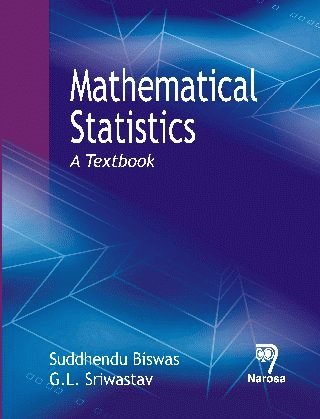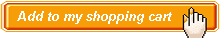Mathematical Statistics:A Textbook Authors:   Suddhendu Biswas, G. L. Sriwastav ISBN: 978-81-7319-927-1 Publication Year:   2011 Pages:   596 Binding:   Paper Back About the book A lucid presentation of modern probability theory based on Measure theoretic approach with examples. Presentation of the genesis of all standard probability distributions (Discrete, Absolutely Continuous and Singular Distributions) inclusive of circular, Singular distributions. New Interpretations of some distributions like Pareto, Logistic etc. Characterization of Distributions and inclusion of Censoring of Distributions is a unique feature of the book. Coverage of Regression Analysis in a text book of statistical methodology is also a new feature of the book. A large number of solved and unsolved examples is also a special feature of the book. Key Features Table of content Preface / Glimpses of Various Aspects of Statistics and History of Statistical Thoughts / Methods of Mathematical Statistics / Probability Theory / Random Variable and Distribution Function / Mathematical Expectation, Convergence of Sequence of Random Variable and Limit Theorems / Specific Distributions – Discrete and Absolutely Continuous / Some Specific Multivariate Distributions / Regression and Correlation / Regression Analysis and its Applications / Characterization of Normal and Other Discrete and Continuous Distribution / Sampling Distributions / Large Sample Theory and Transformations in Statistics / Theory of Estimation / Testing of Statistical Hypothesis / Appendix / Bibliography / Index.Audience Undergraduate - Postgraduate Students & Researchers